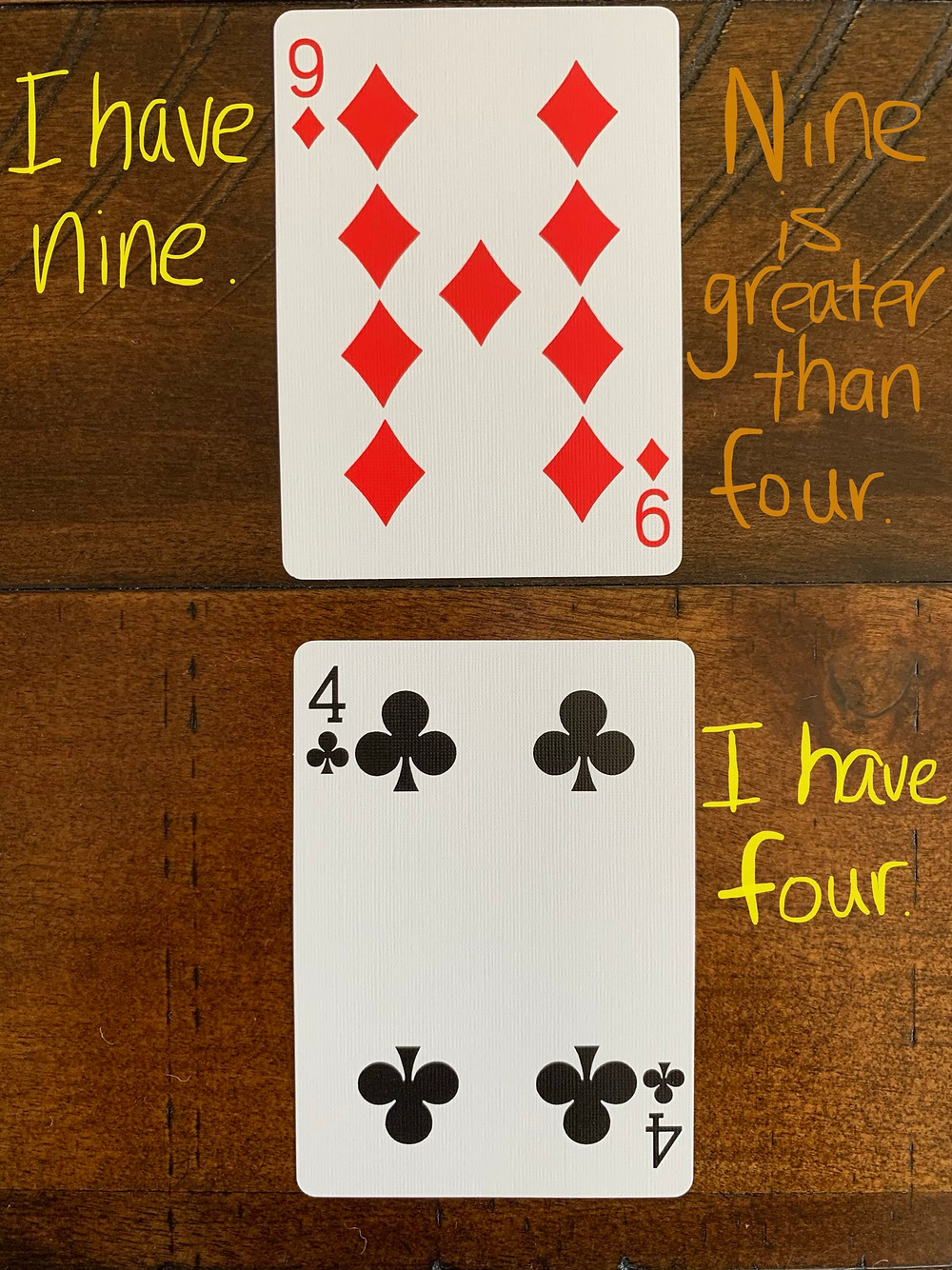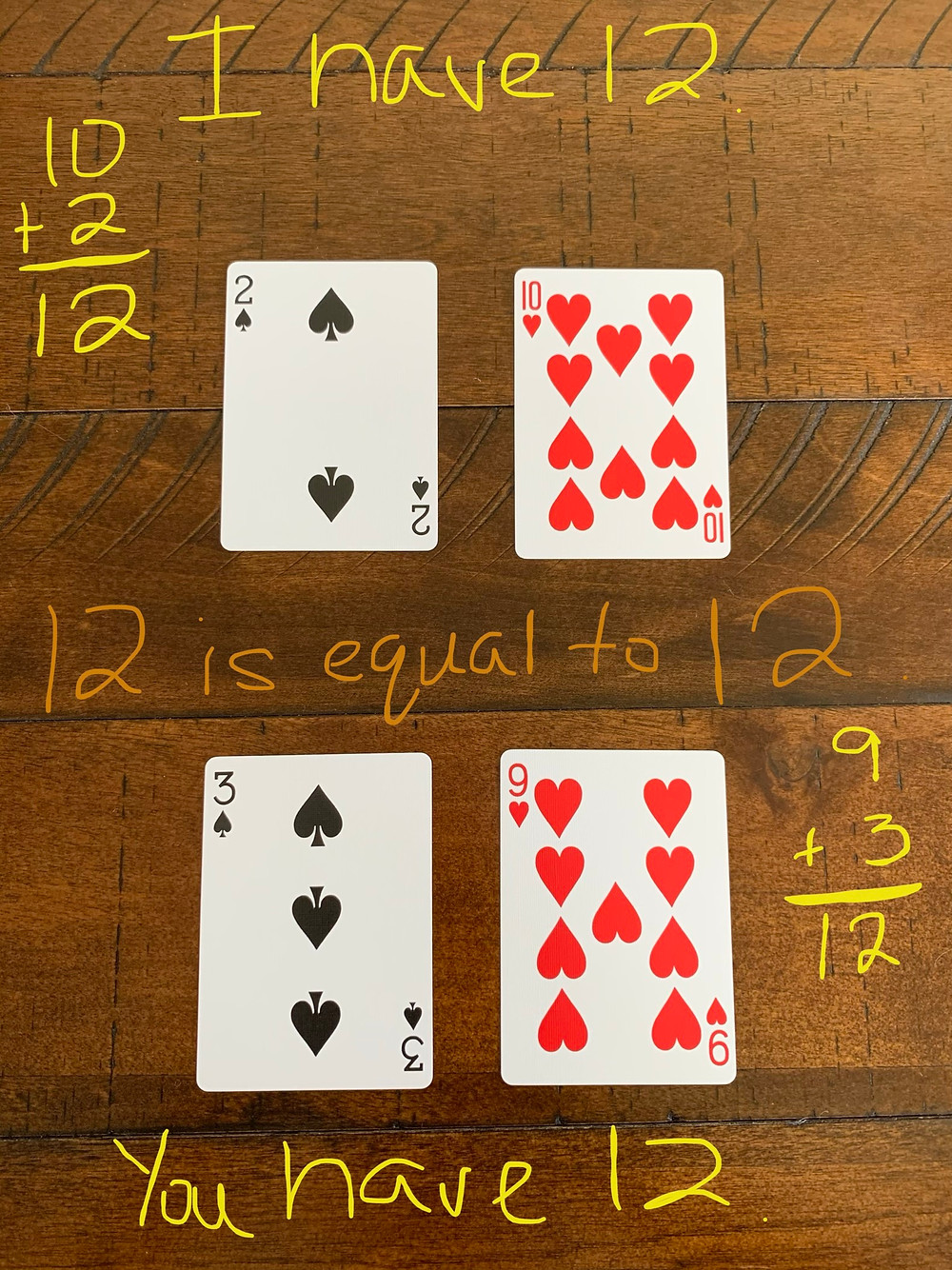Search

# You Want to Engage Your Students? Play Card Games!

Kids love math games that involve playing cards. The mystery of shuffling and then anticipating which cards will appear is part of the fun. And games that require students to make decisions and use their reasoning and problem solving skills can help develop their number sense.

In this blog post, we’ll explore card games across the grade levels that are easy to implement whether you are teaching remotely or face to face. If you are teaching remotely, you can use this link https://deck.of.cards that provides you and your students with a virtual card deck.

The following games are engaging, motivating, and create contexts for students to talk about their math reasoning. For each card game, there is a set of easy-to-follow directions and a list of things teachers should look for as they observe their students play.

You can use the games as a whole class routine, or you can model the game and then have partners or small groups play. These card games are a great way to address math standards from preschool and beyond! We’ve borrowed some of the ideas for these card games from two wonderful math resources, Math Solutions and Investigations

*Note: When using playing cards, we recommend taking out the face cards. Aces are equal to 1. If you want to challenge the students, you can make the Queen wild (a player can make the Queen equal any number, 1-10).

### How Many Do You See? How Do You See Them?How to Play:

If you are teaching remotely, you can take a screenshot of a photo of a playing card, leaving off the numbers and extra figures. If you are teaching in person, cover up the numbers and extra figures. Show the students the card and simply ask, “How many do you see? How do you see them?”

What to Look For:

•How do students figure the total?

•Do they count by ones? Are they able to correctly count each figure without double counting (one-to-one correspondence)?

•Are students able to figure the correct total?

•Are students able to figure the total by seeing groups of figures (“I see five and I see three,” or, “I see four groups of two”).

### CompareHow to Play:

1. Have students play in pairs.

2. Shuffle the cards.

3. Each player takes a card and turns it over.

4. The players compare the numbers.

5. Players take turns using the following frame: I have ____. You have ___. ___ is greater than/equal to ___.

6. The player with the greater number takes the cards. If the numbers are equal, the players play again. Whoever has the greater amount takes all of the cards.

7. Continue playing.

What to Look For:

•How do students figure the total?

•Do they count by ones? Are they able to correctly count each figure without double counting (one-to-one correspondence)?

•Are students able to figure the correct total?

•Are students able to figure the total by seeing groups of figures (“I see five and I see three,” or, “I see four groups of two”).

•Are students able to figure out which number is greater than or equal to the other? How do they figure this out?

•Are students able to use the sentence frames to describe and compare the amounts?

### Double CompareHow to Play:

1. Have students play in pairs.

2. Shuffle the cards.

3. Each player takes two cards and turns them over.

4. The players add the numbers together to find the sum.

5. Players take turns using the following frame: I have ___. You have ___. ____ is great than/equal to ___.

6. The player with the greater sum takes the cards. If the sums are equal, the players play again. Whoever has the greater sum takes all of the cards.

7. Continue playing.

What to Look For:

•How does each player figure the total?

•Do they use the figures and count all? Do they count on? Do they use number sense strategies? ( e.g. 8 + 7:  “I broke apart 7 into 5 + 2; 8 + 2 is 10, and 10 + 5 is 15”).

•Do they immediately know the sum?

•Are students able to use the sentence frames to describe and compare the amounts?

### Close to 20How to Play:

1. Have students play in pairs or groups of 3 or 4.

2. Shuffle the cards.

3. Each player takes five cards (if face cards appear, disregard) and turns them over.

4. Each player chooses three of the five cards to add together to get a sum closest to or equal to 20 (they can go over or under 20).

5. A player’s score for each round is how far away from 20 they are (e.g. if they chose cards with 3, 10, and 5, the sum is 18. Their score for the round is 2).

6. After a round, players reshuffle the card deck and continue play.

7. Players play five rounds and then add up their scores. The player with the lowest score wins!

What to Look For:

How do students decide which three cards to choose? Do they have a sense of how close they will be to 20 when choosing the cards?

•Are students able to explain their reasoning for the decisions they make?

•How do students combine the three numbers they choose?

•Do they use the figures and count all? Do they count on? Do they use number sense strategies? ( e.g. 8 + 7:  “I broke apart 7 into 5 + 2; 8 + 2 is 10, and 10 + 5 is 15”).

•How close to 20 do students get when finding the sum of their three numbers?

### Close to 100How to Play:

1. Have students play in pairs or groups of 3 or 4.

2. Shuffle the cards.

3. Each player takes six cards (if face cards or ten cards appear, disregard) and turns them over.

4. Each player chooses four of the six cards and creates a two-digit plus two-digit problem; players try to get a sum closest to 100   (e.g. a player takes the following cards:  3, 5, 8, 7, 2, 3. They choose to create the problem 83 + 23=106).

5. A player’s score for each round is how far away from 100 they are (e.g. 83 + 23= 106; the player’s score for that round is 6).

6. After a round, players reshuffle the card deck and continue play.

7. Players play five rounds and then add up their scores. The player with the lowest score wins!

What to Look For:

•How do students decide which four cards to choose? Do they have a sense of how close to 100 they will be when they choose the cards?

•Are students able to explain their reasoning for the decisions they make?

•How do students combine the quantities in the problem they set up?

•When adding the numbers together, do students start in the ten’s place, beginning with the biggest quantities or do they start adding in the one’s place?

-Do students showcase their understanding of place value by referring to the digits as the quantities they represent? For example, when a player adds together 65 + 28, they might say, “Sixty plus twenty is 80” rather than, “Six plus two is 8.”

### Close to 1000How to Play:

1. Have students play in pairs or groups of 3 or 4.

2. Shuffle the cards.

3. Each player takes eight cards (if face cards or ten cards appear, disregard) and turns them over.

4. Each player chooses six of the eight cards and creates a three-digit plus three-digit problem; players try to get a sum closest to 1000 (e.g. a player takes the following cards:  3, 5, 8, 2, 2, 3, 9, 1. They choose to create the problem 132 + 925=1,057).

5. A player’s score for each round is how far away from 1000 they are (e.g. 132 + 925 =1,057; the player’s score for that round is 57).

6. After a round, players reshuffle the card deck and continue play.

7. Players play five rounds and then add up their scores. The player with the lowest score wins!

What to Look For:

•How do students decide which six cards to choose? Do they have a sense of how close to 1000 they will be when they choose the cards?

•Are students able to explain their reasoning for the decisions they make?

•How do students combine the quantities in the problem they set up? Are they easily able to compute mentally?

•When adding the numbers together, do students start in the hundreds place, beginning with the biggest quantities or do they start adding in the one’s place?

-Do students showcase their understanding of place value by referring to the digits as the quantities they represent? For example, when a player adds together 132 + 925, they might say, “Nine hundred  plus one hundred is one thousand” rather than, “Nine plus one is ten.”

### Integer Card Game

How to Play:

1. Have students play in pairs or groups of 3 or 4.

2. Shuffle cards.

3. Each player takes four cards and turns them over.

4. Red cards are negative and black cards are positive.

5. After dealing, turn the card on the top of the deck face up next to the pile. This will serve as the discard pile.

6. Players take turns. On their turn, a player can either take the top card on the discard pile or from the top of the deck. The player MUST keep this card and discard a card from their hand.

7. Play continues with the next player until all players have picked and discarded four times.

8. Players add the four cards together and the player with a score of zero or the closest to zero wins the round (see an example of a round below).What to Look For:

•How do students decide which cards to choose? Do they have a sense of how close to zero they will be when choosing the cards?

•Are students able to explain their reasoning for the decisions they make?

•How do students figure the answer to the problem they finally set up?

•Can students reason mentally or do they need supports such as a number line?

•Do students realize that no matter how you order the cards, the result will be the same (associative property of addition)?